# The Complete List of Homeschool Curriculum

Each of our curriculum has been reviewed by a homeschool parent.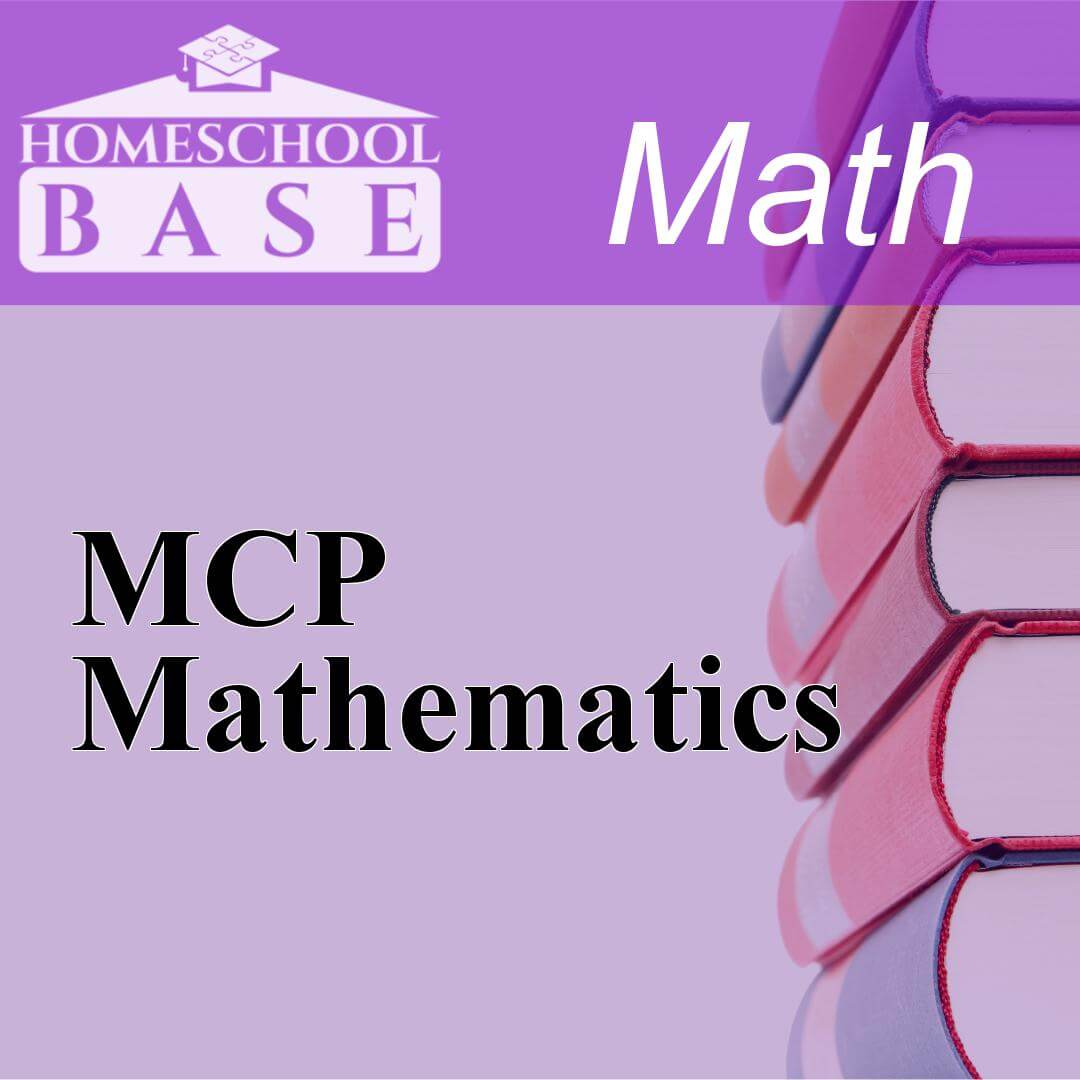## MCP Mathematics

MCP Mathematics is a Math curriculum for grades K-6. This program teaches by using the sequential method. The average amount of teacher involvement on the parent(s) is: medium. This curriculum does no...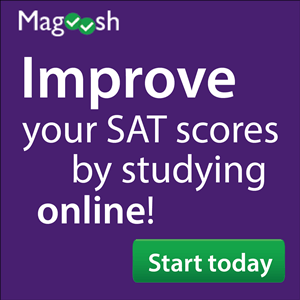## Magoosh SAT Test Prep

Taking the SAT and feeling a little unprepared? Magoosh is your best option for online test prep! 100s of videos and practice questions and a GUARANTEED score increase....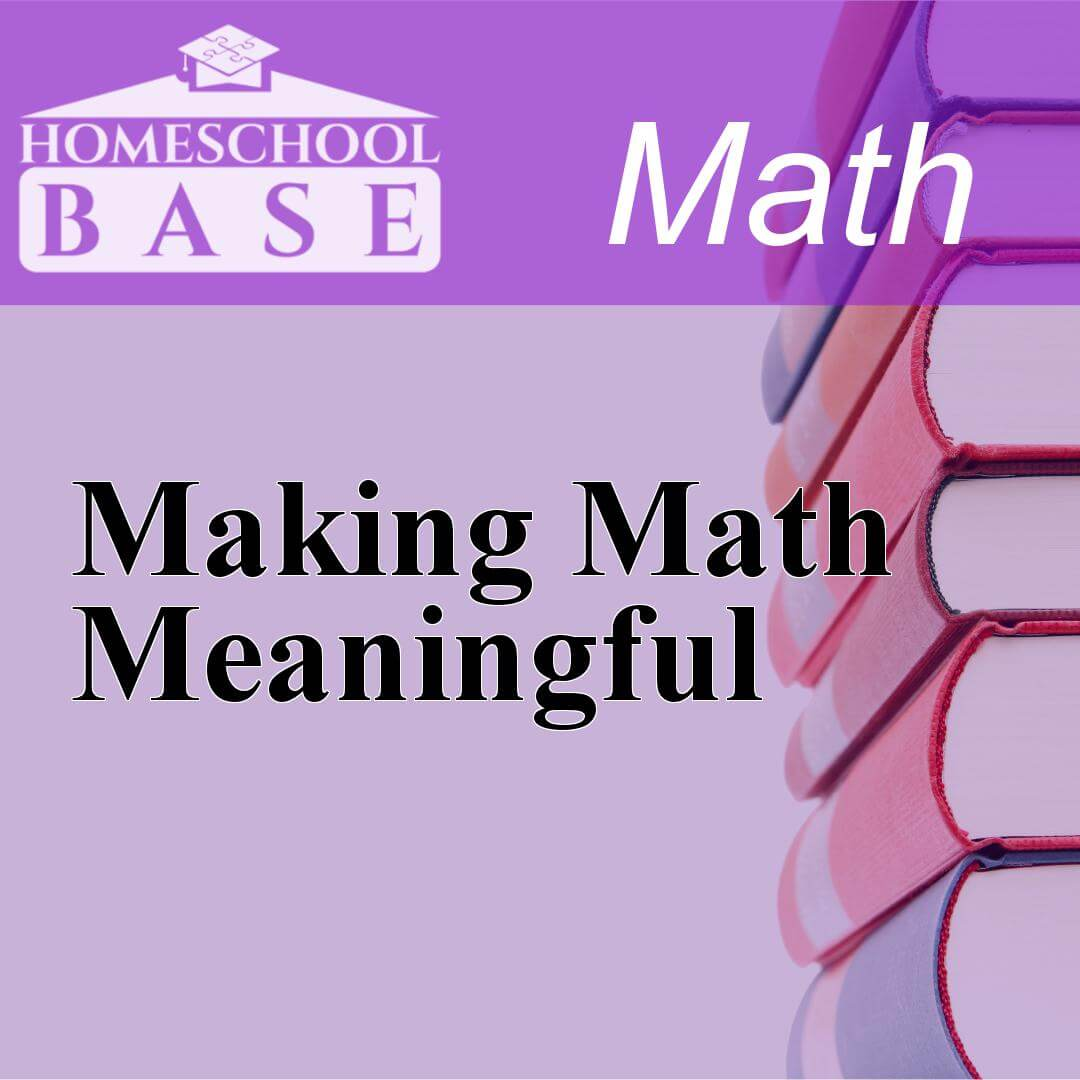## Making Math Meaningful

Making Math Meaningful is a Math curriculum for grades K-8. This program teaches by using the conceptual-topical method. The average amount of teacher involvement on the parent(s) is: high. This curri...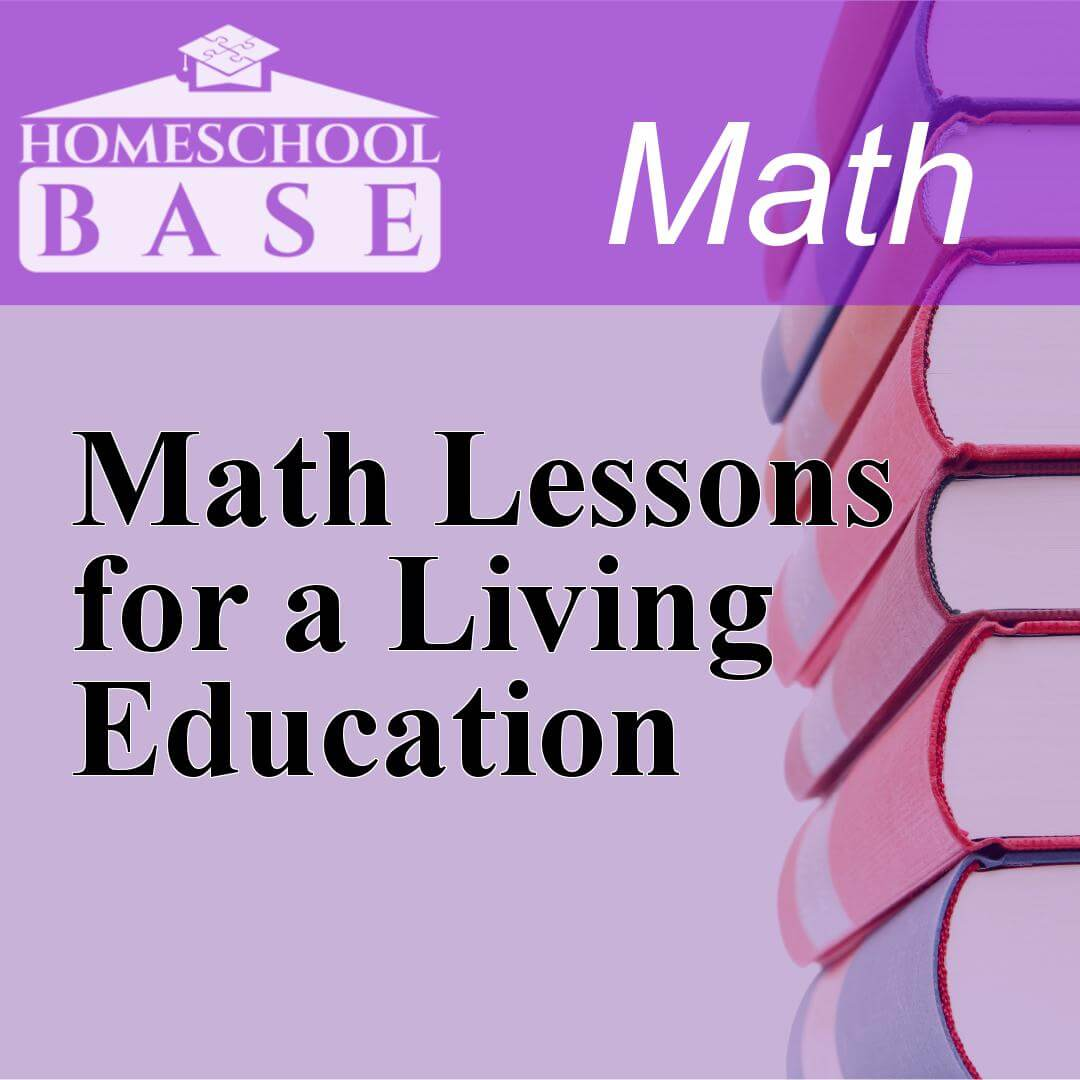## Math Lessons for a Living Education

Math Lessons for a Living Education is a Math curriculum for grades PK-5. This program teaches by using the conceptual-topical method. The average amount of teacher involvement on the parent(s) is: hi...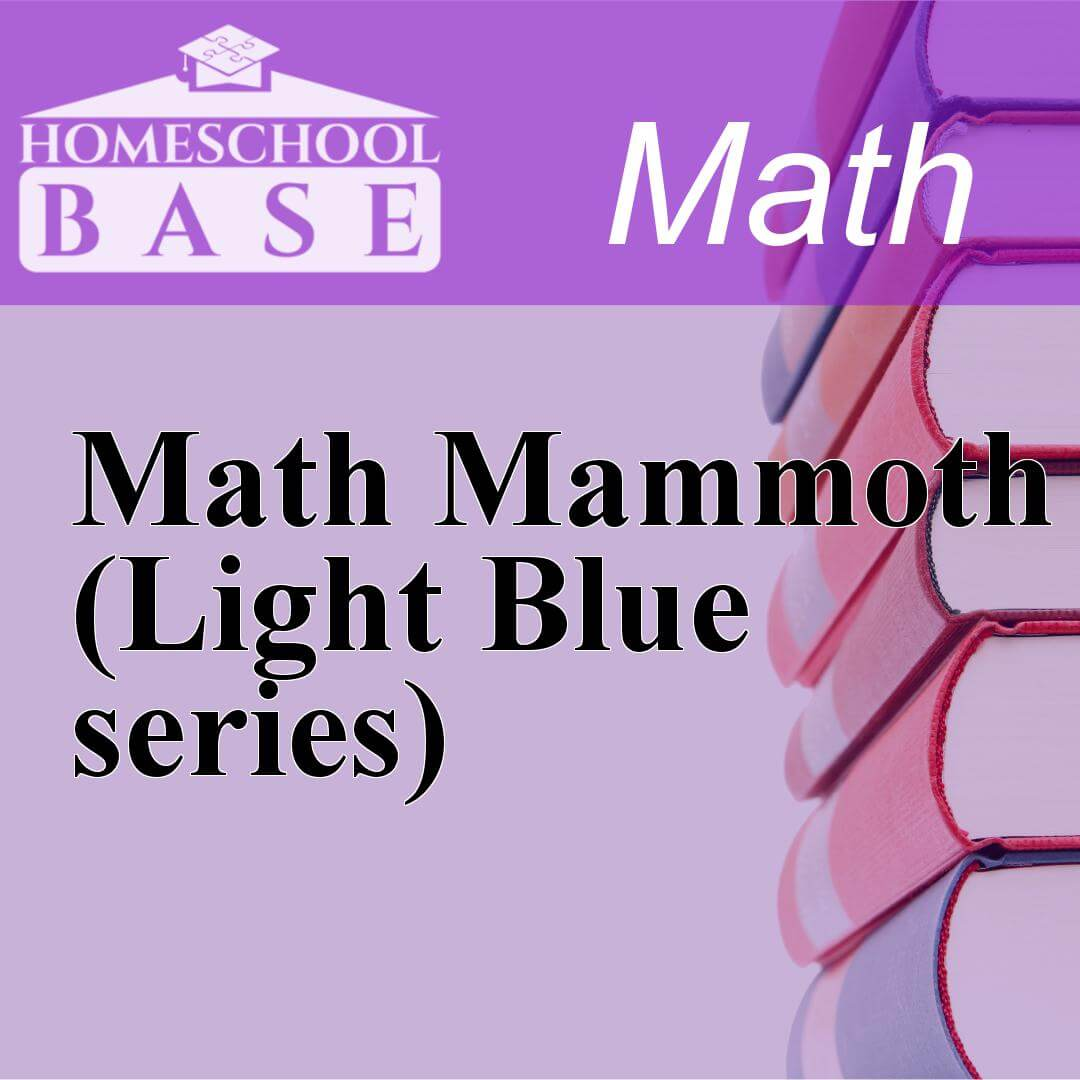## Math Mammoth (Light Blue series)

Math Mammoth (Light Blue series) is a Math curriculum for grades 1-7. This program teaches by using the sequential method. The average amount of teacher involvement on the parent(s) is: low. This cur...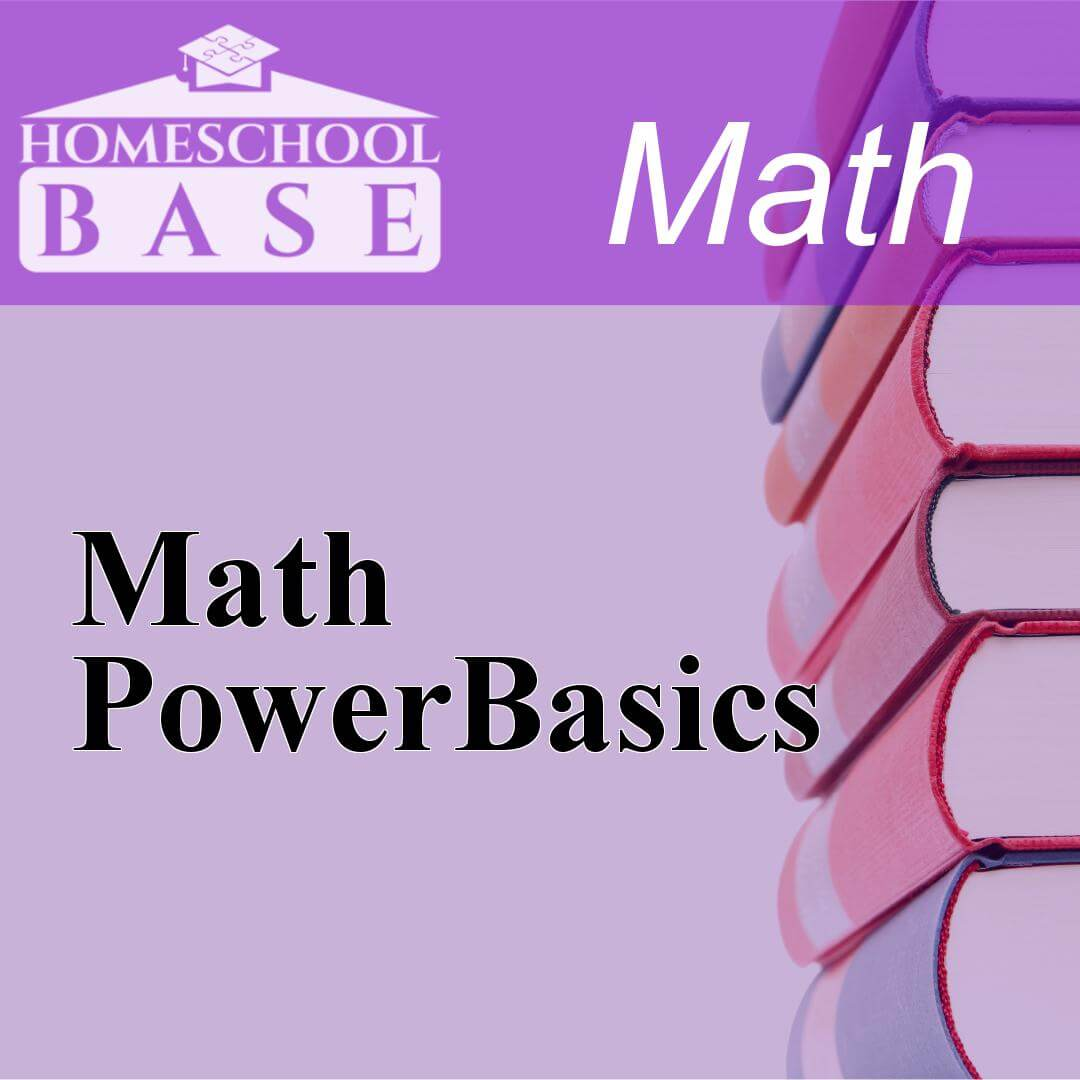## Math PowerBasics

Math PowerBasics is a Math curriculum for grades 6-12. This program teaches by using the sequential method. The average amount of teacher involvement on the parent(s) is: low. This curriculum does not...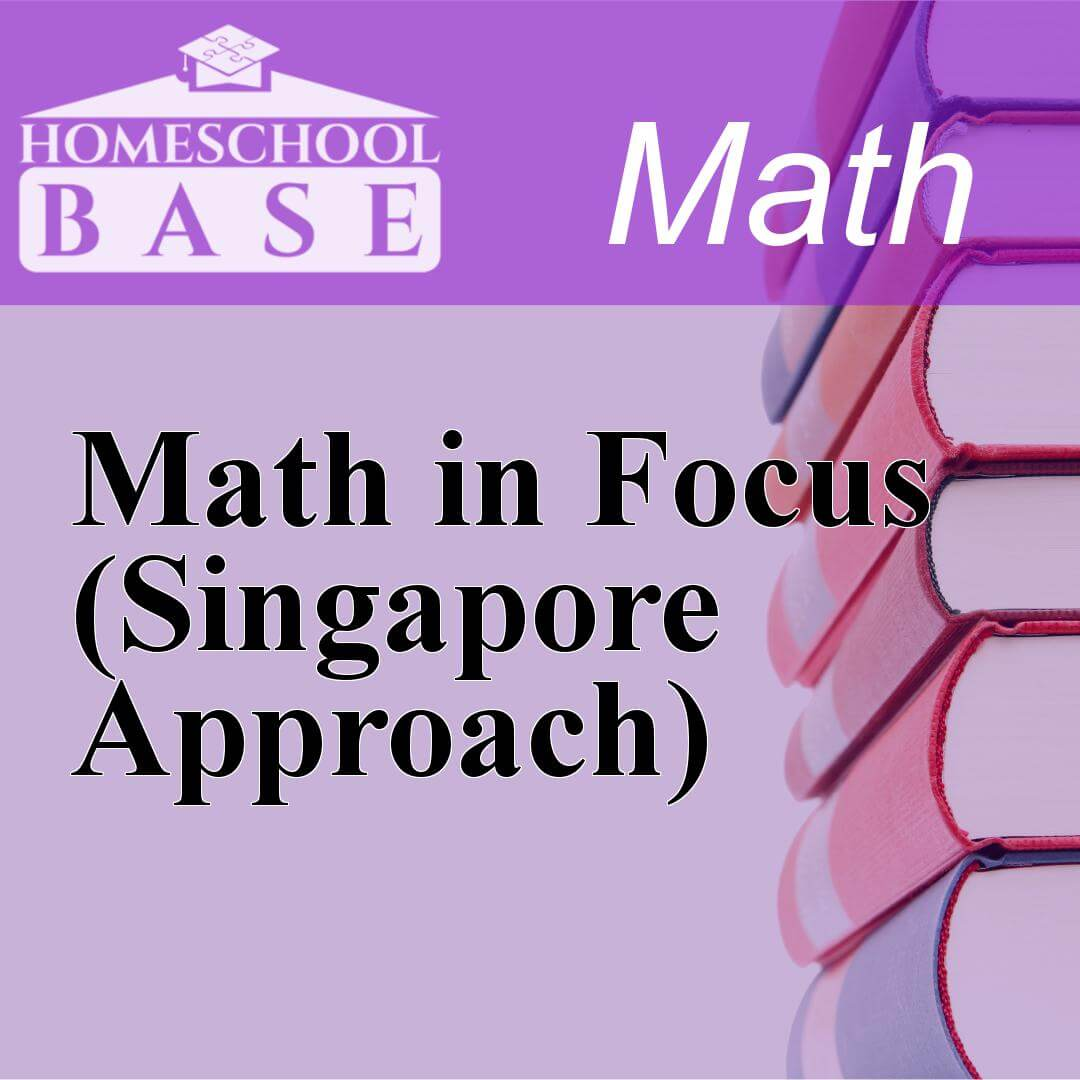## Math in Focus (Singapore Approach)

Math in Focus (Singapore Approach) is a Math curriculum for grades K-8. This program teaches by using the sequential method. The average amount of teacher involvement on the parent(s) is: medium. Thi...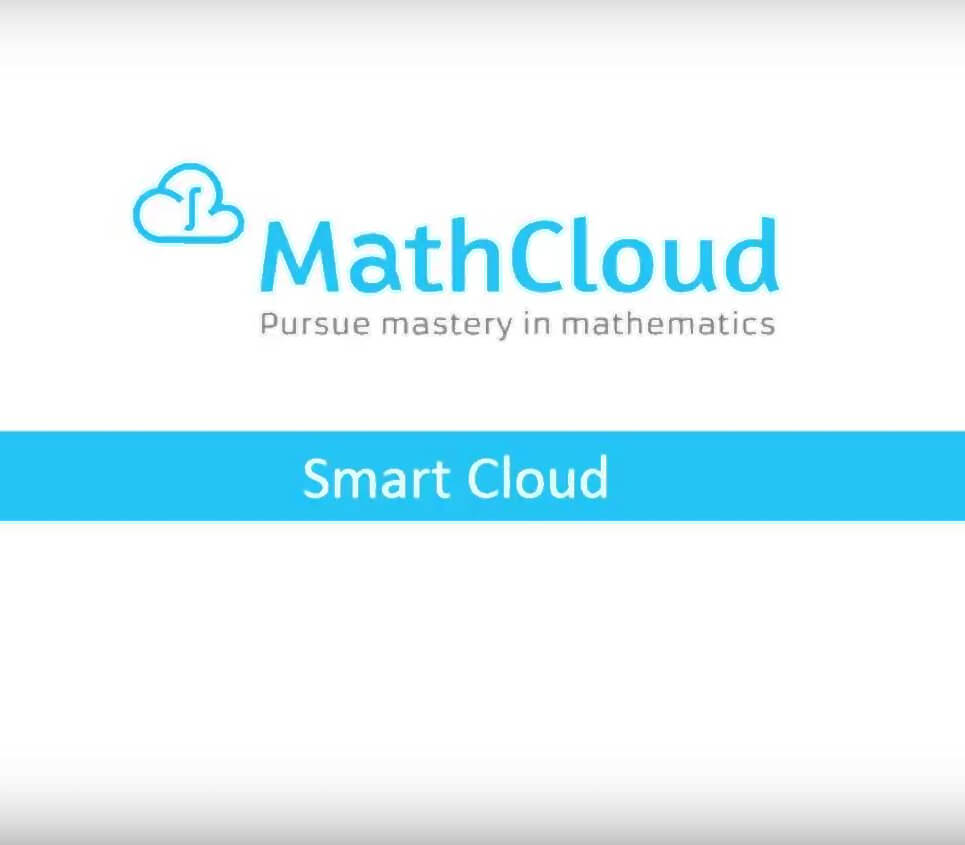## MathCloud

MathCloud offers a new approach to learning mathematics. Rather than directing students to memorize solutions and techniques, MathCloud teaches students to break complex problems down into parts and u...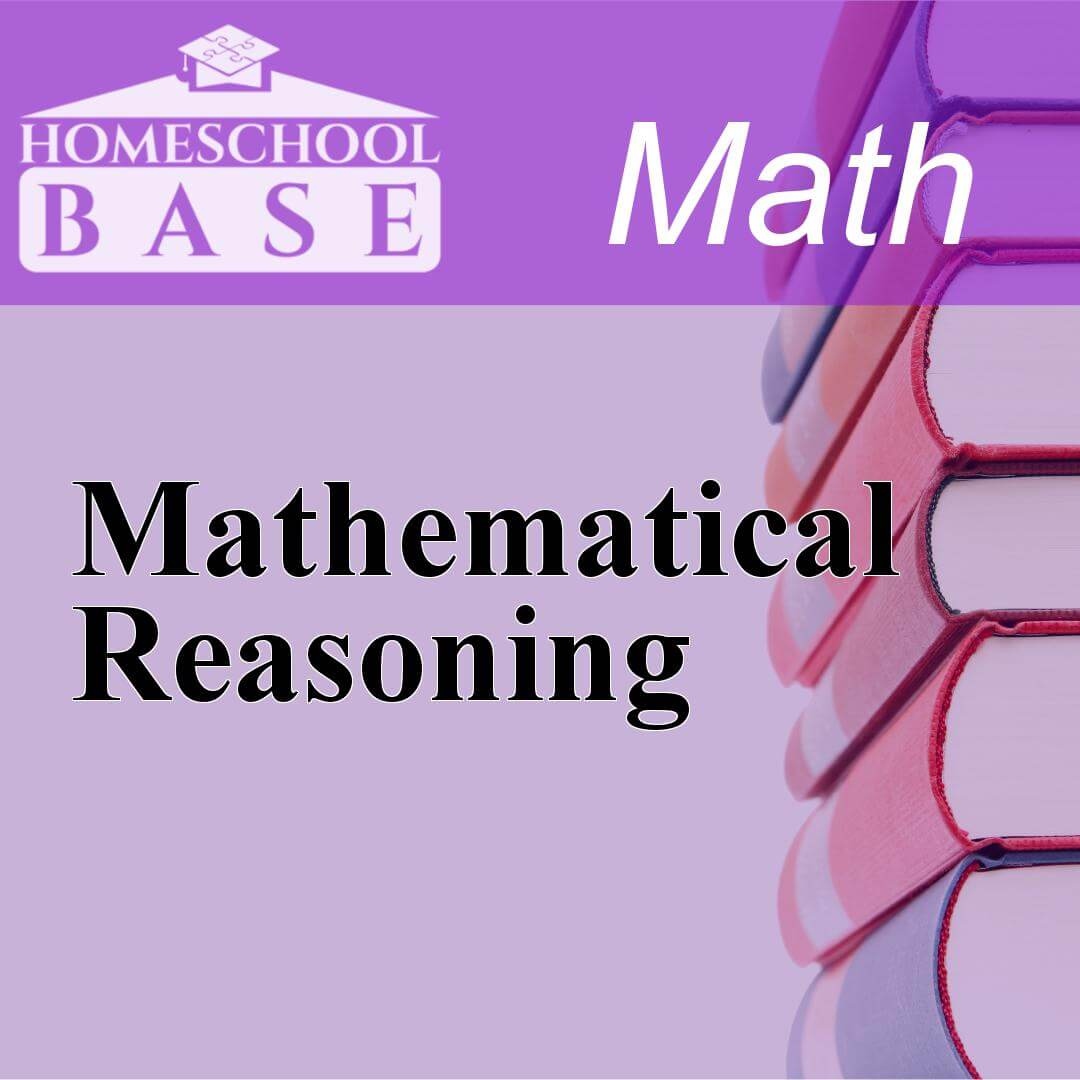## Mathematical Reasoning

Mathematical Reasoning is a Math curriculum for grades PK-9. This program teaches by using the spiral method. The average amount of teacher involvement on the parent(s) is: low. This curriculum does n...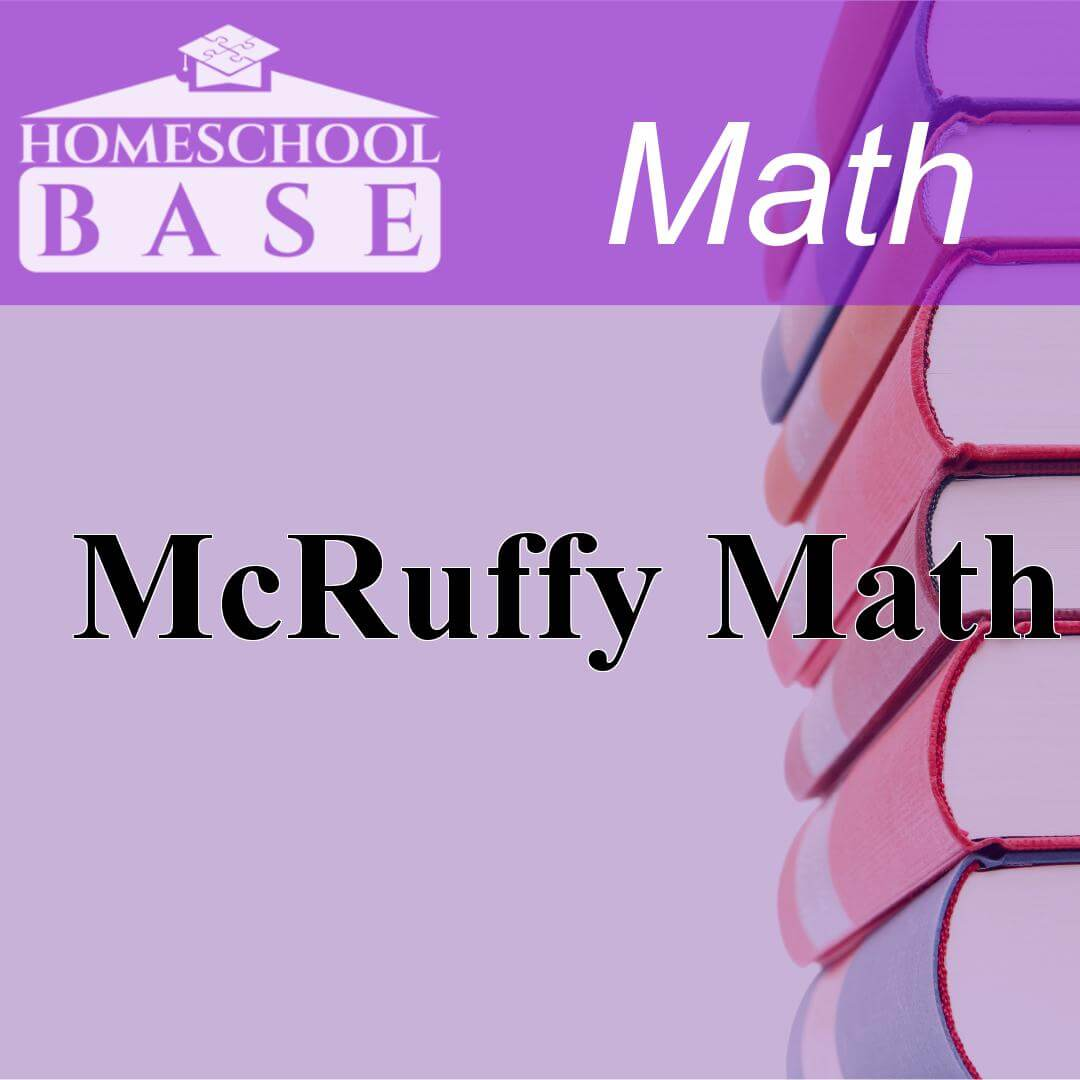## McRuffy Math

McRuffy Math is a Math curriculum for grades K-3. This program teaches by using the spiral method. The average amount of teacher involvement on the parent(s) is: medium. This curriculum does not confo...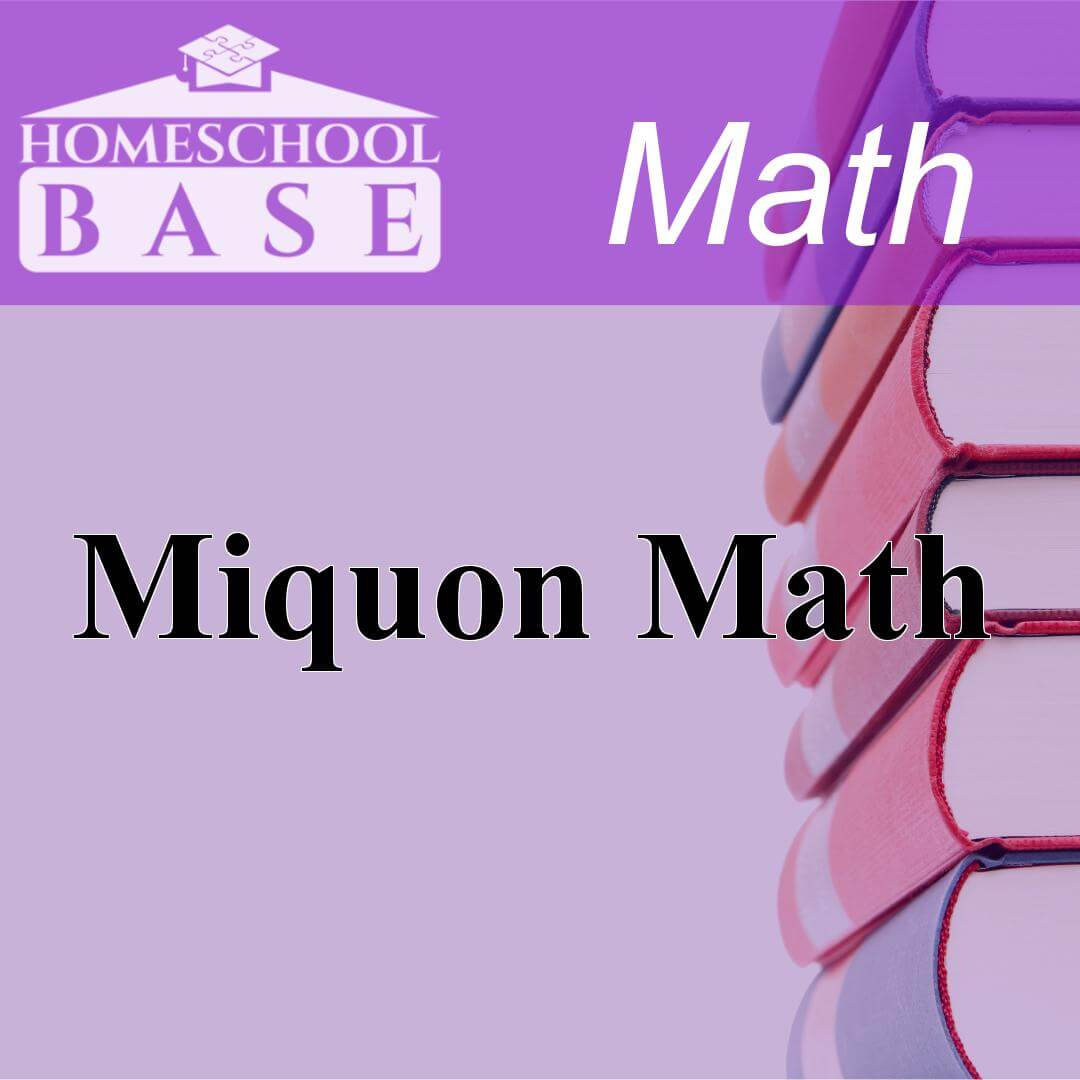## Miquon Math

Miquon Math is a Math curriculum for grades 1-3. This program teaches by using the conceptual-topical method. The average amount of teacher involvement on the parent(s) is: high. This curriculum does ...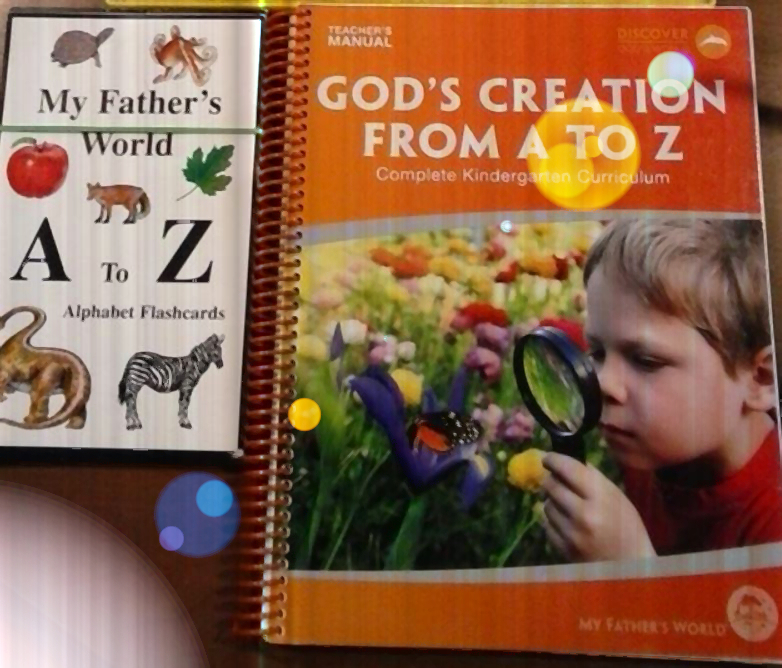## My Father's World

My Father’s World is a complete grade-level curriculum for preschool through eighth grade and offers almost-complete curriculum through twelfth grade. My Father’s World is inspired by several homescho...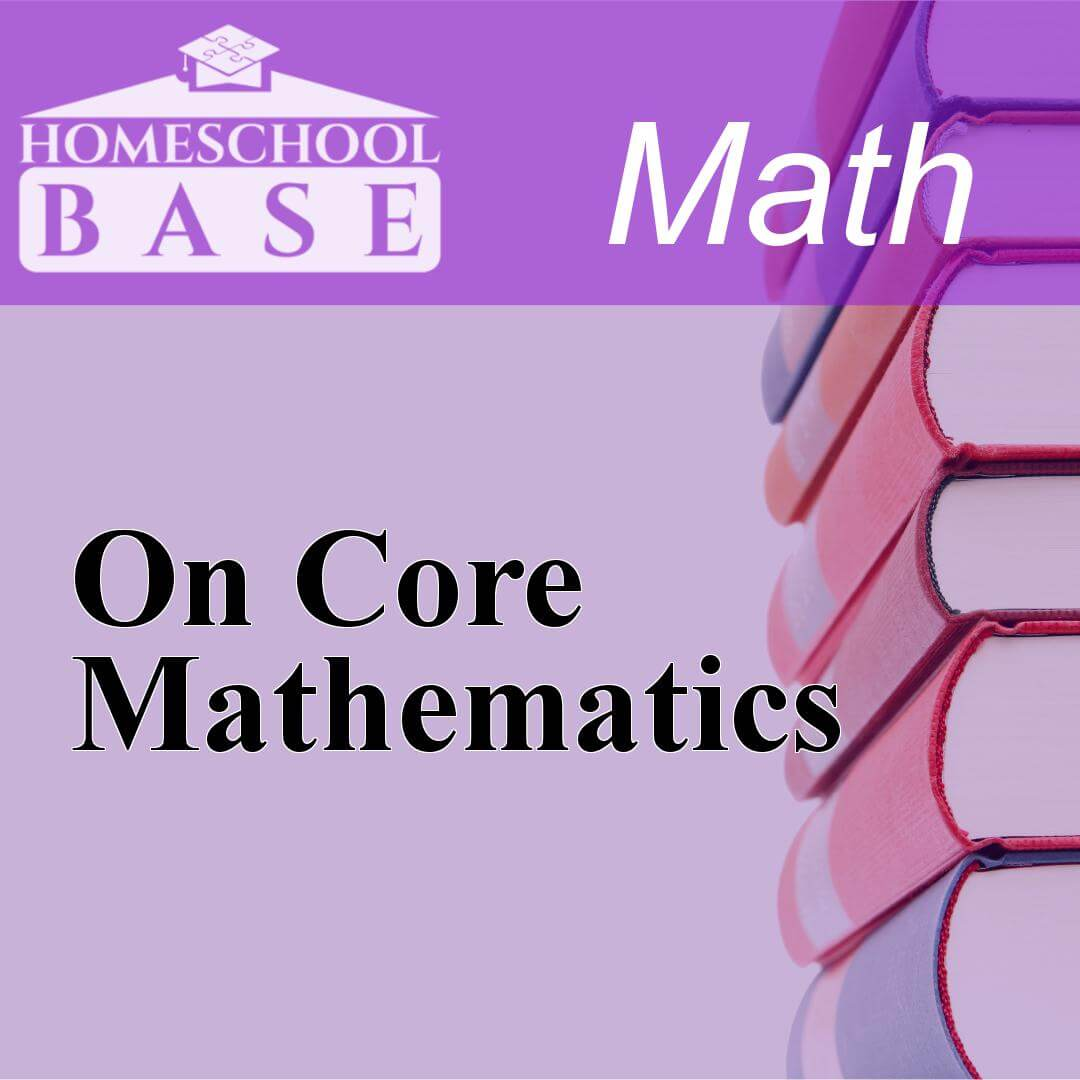## On Core Mathematics

On Core Mathematics is a Math curriculum for grades K-12. This program teaches by using the conceptual-topical method. The average amount of teacher involvement on the parent(s) is: medium. This curri...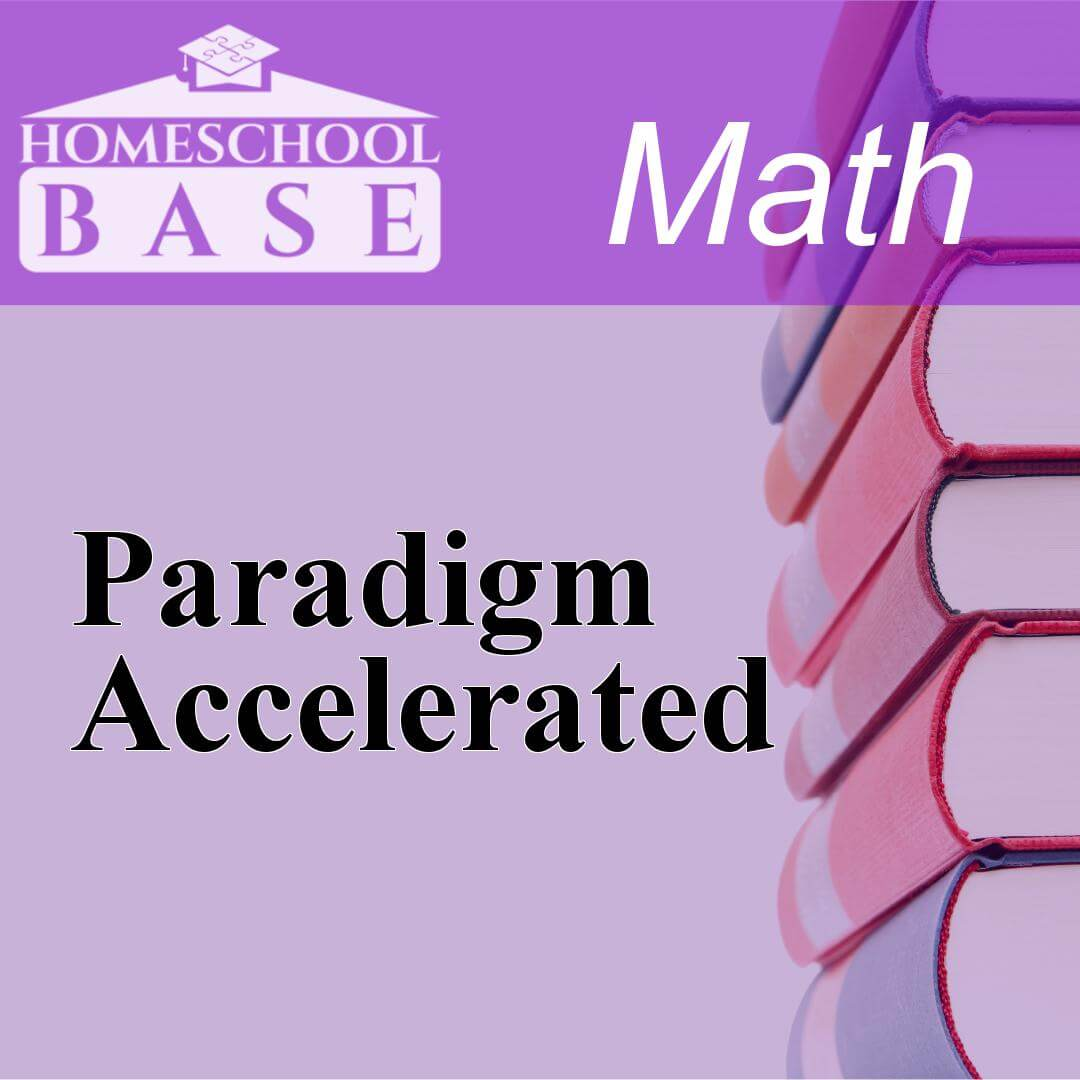Paradigm Accelerated is a Math curriculum for grades 7-8. This program teaches by using the sequential method. The average amount of teacher involvement on the parent(s) is: low. This curriculum does ...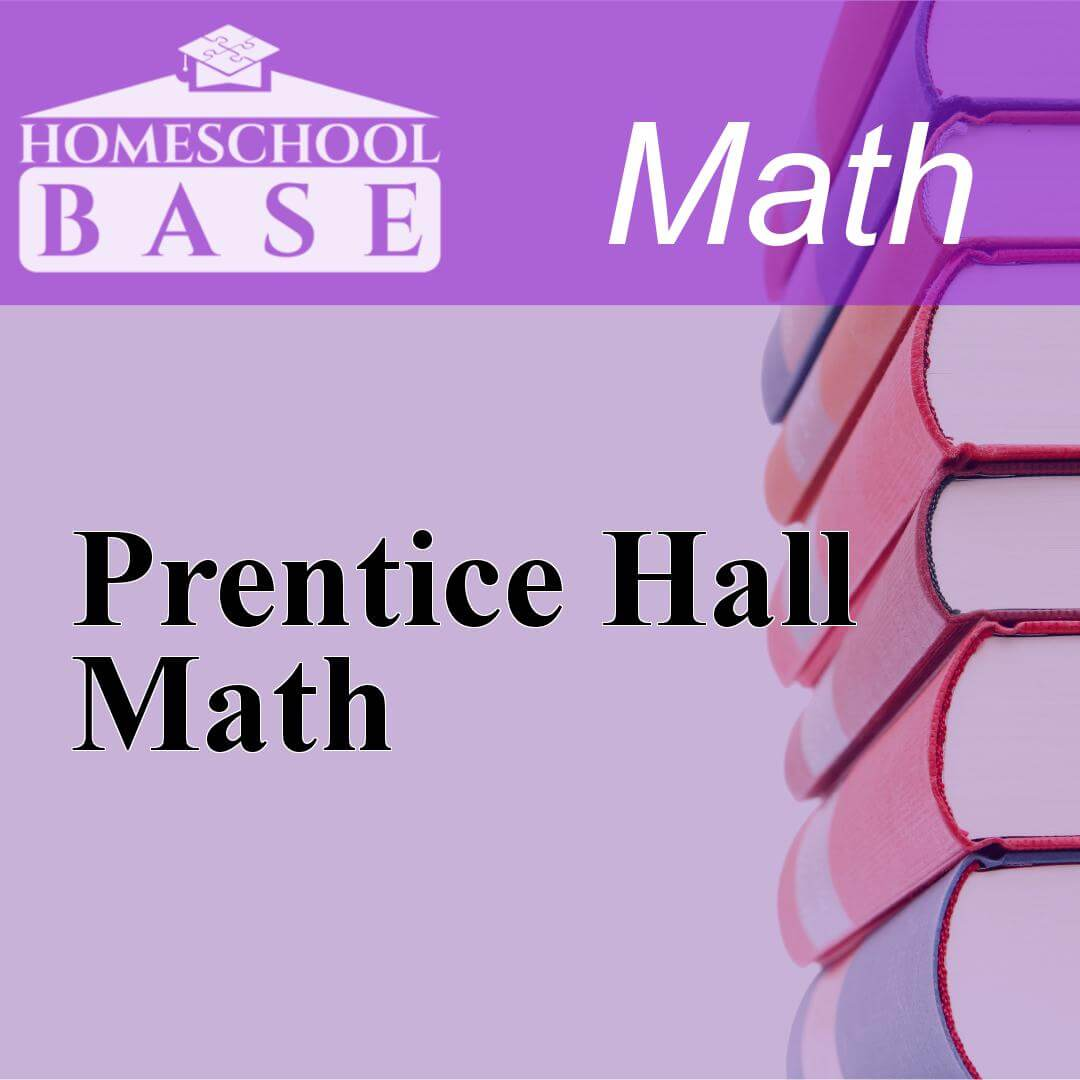## Prentice Hall Math

Prentice Hall Math is a Math curriculum for grades 6-10. This program teaches by using the sequential method. The average amount of teacher involvement on the parent(s) is: medium. This curriculum doe...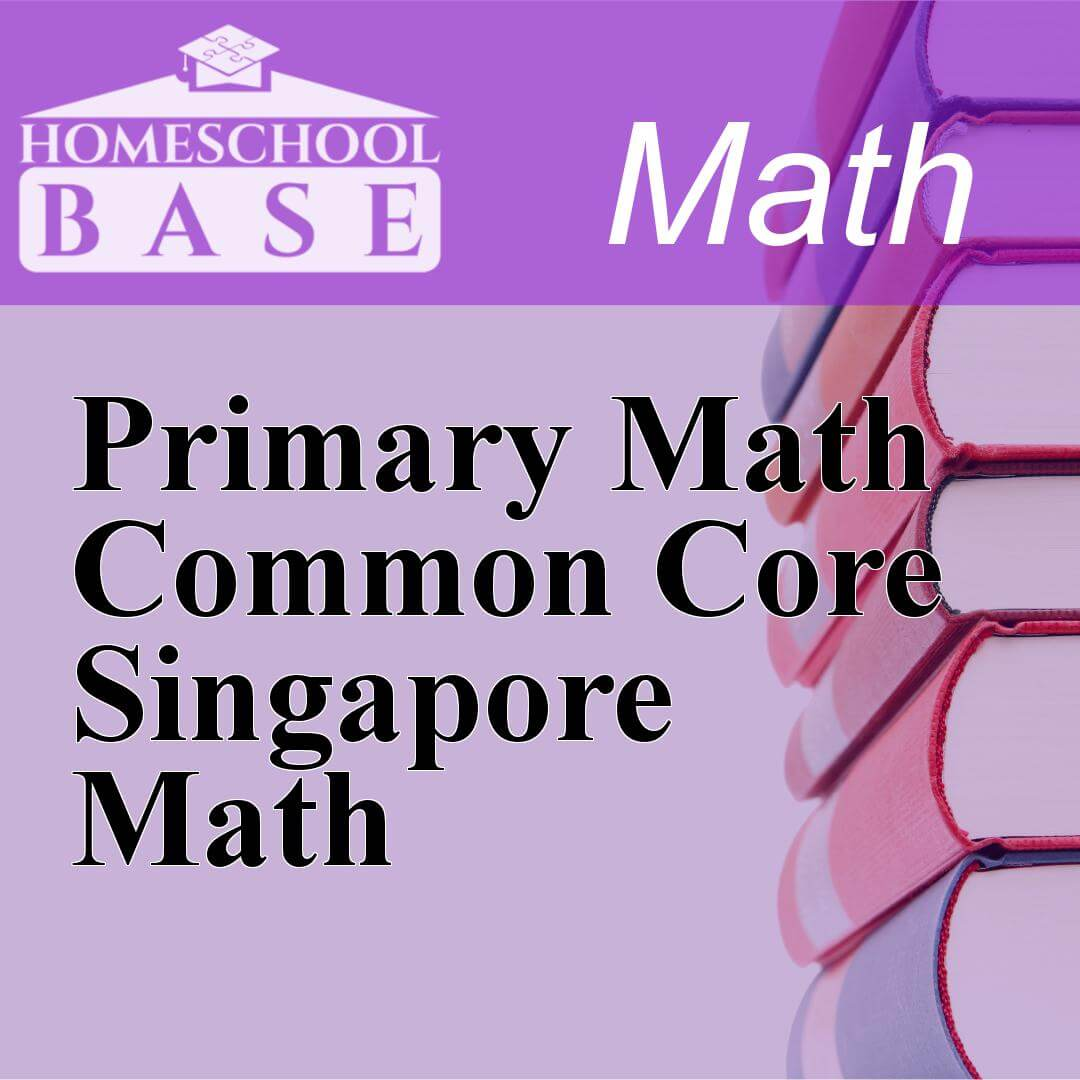## Primary Math Common Core Singapore Math

Primary Math Common Core Singapore Math is a Math curriculum for grades K-5. This program teaches by using the sequential method. The average amount of teacher involvement on the parent(s) is: medium....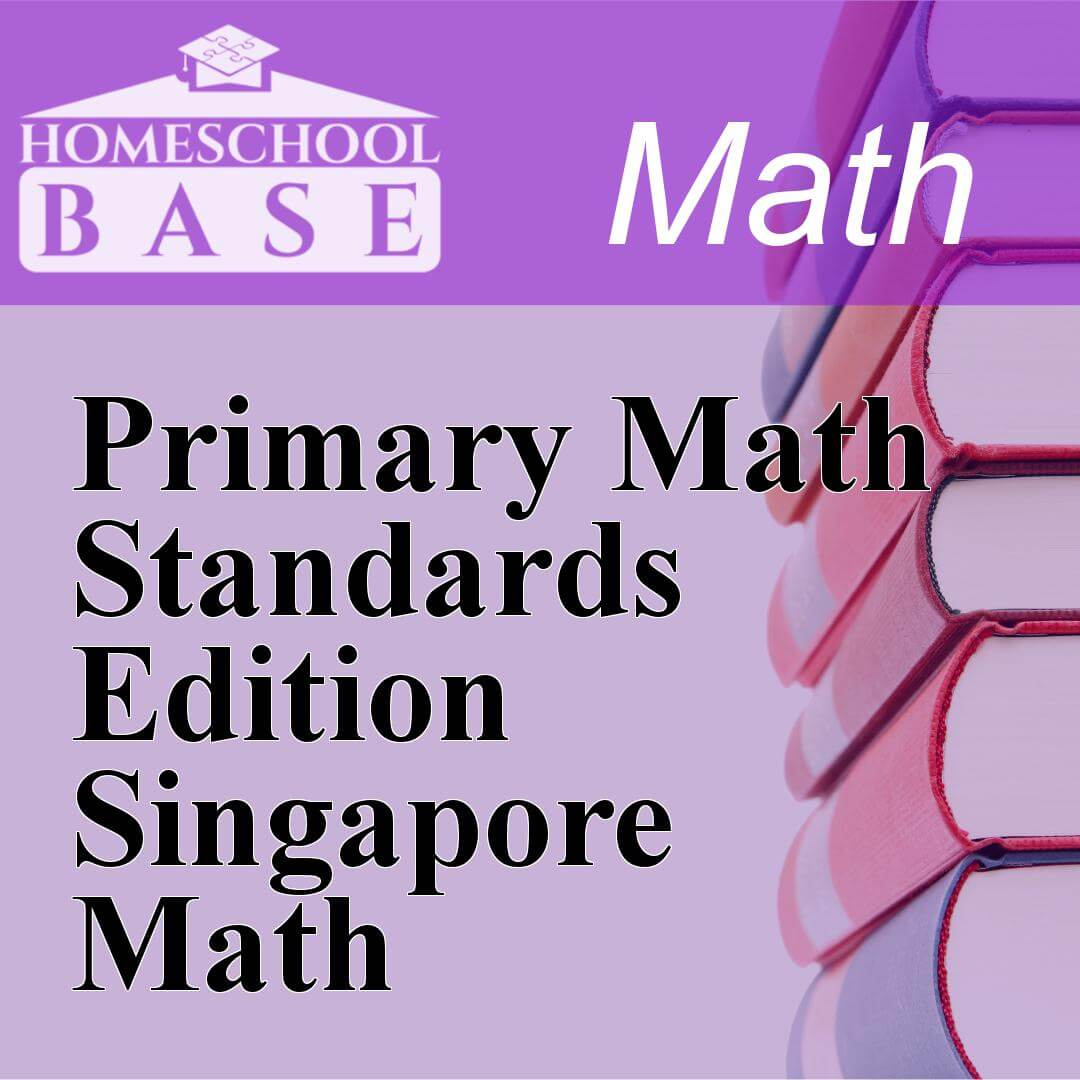## Primary Math Standards Edition Singapore Math

Primary Math Standards Edition Singapore Math is a Math curriculum for grades K-6. This program teaches by using the sequential method. The average amount of teacher involvement on the parent(s) is: m...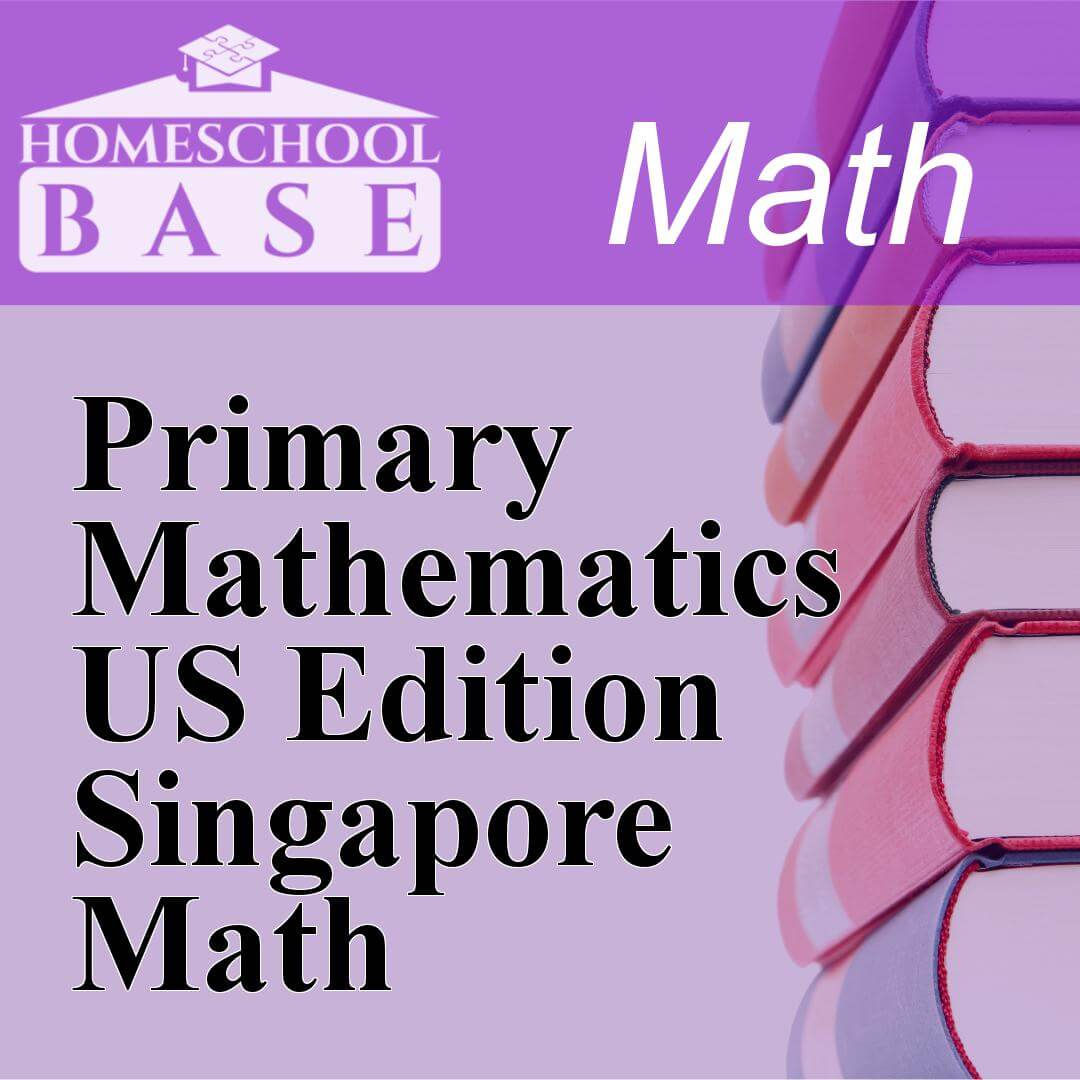## Primary Mathematics US Edition Singapore Math

Primary Mathematics US Edition Singapore Math is a Math curriculum for grades K-6. This program teaches by using the sequential method. The average amount of teacher involvement on the parent(s) is: ...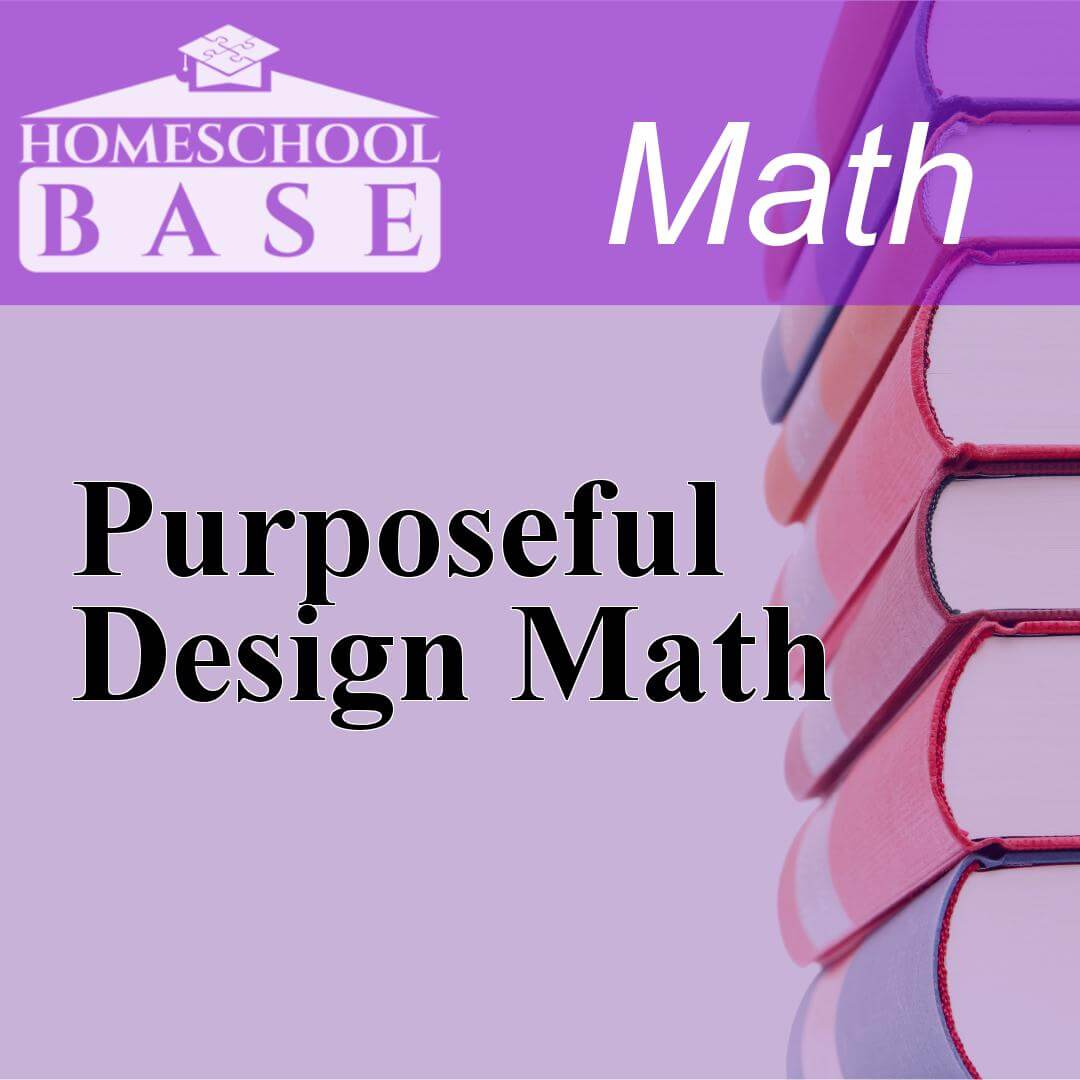## Purposeful Design Math

Purposeful Design Math is a Math curriculum for grades K-8. This program teaches by using the sequential method. The average amount of teacher involvement on the parent(s) is: high. This curriculum do...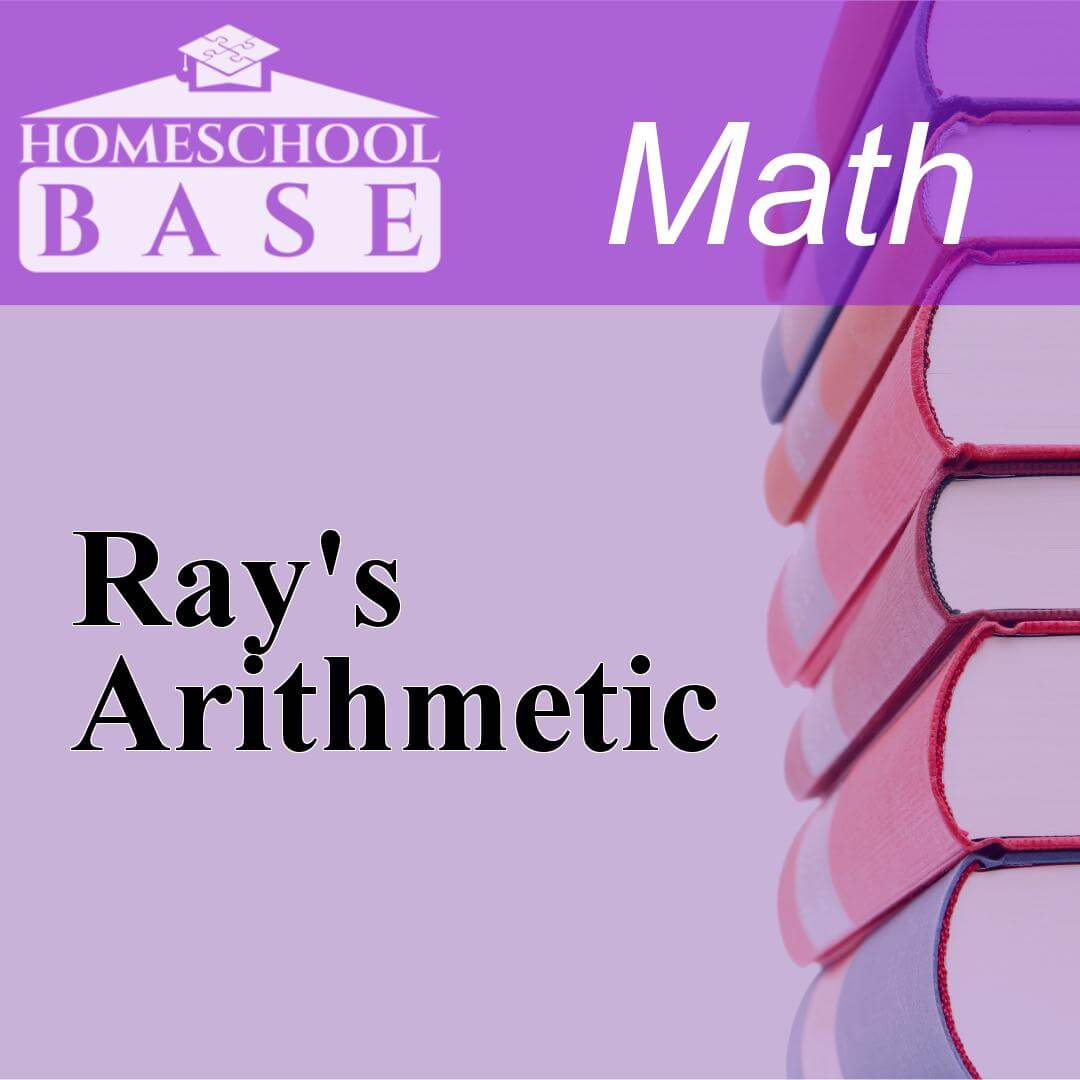## Ray's Arithmetic

Ray's Arithmetic is a Math curriculum for grades 1-8. This program teaches by using the sequential method. The average amount of teacher involvement on the parent(s) is: medium. This curriculum does n...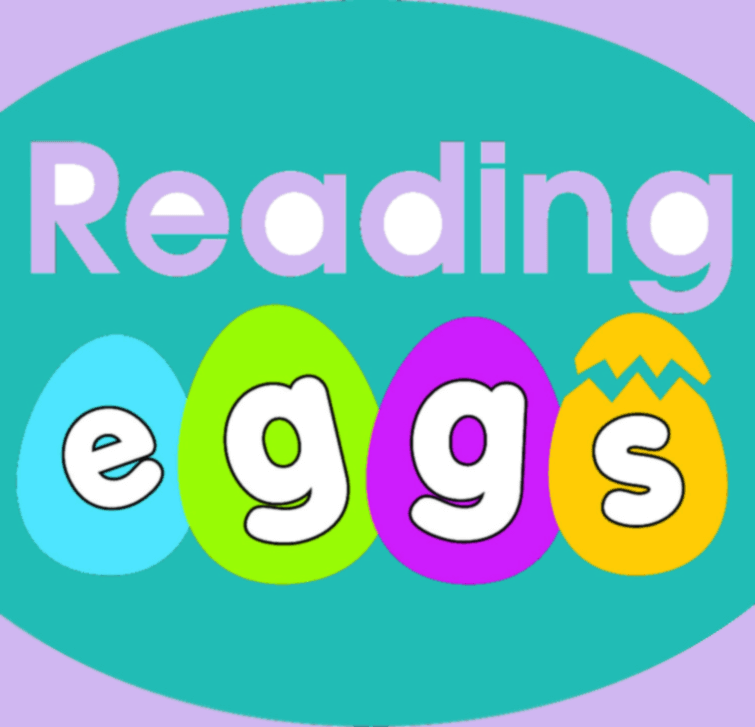Reading Eggs is a multi award-winning online reading program that focuses on a core reading curriculum of skills and strategies essential for sustained reading success....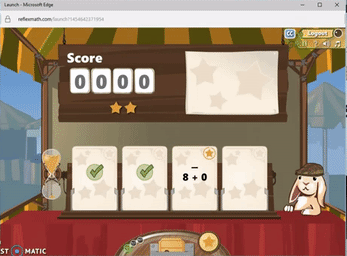## Reflex Math

Read our review of the best online math drill program and save 44% on a subscription! Reflex Math publishes high quality games that children really do enjoy playing. Reflex Math does a surprisingly go...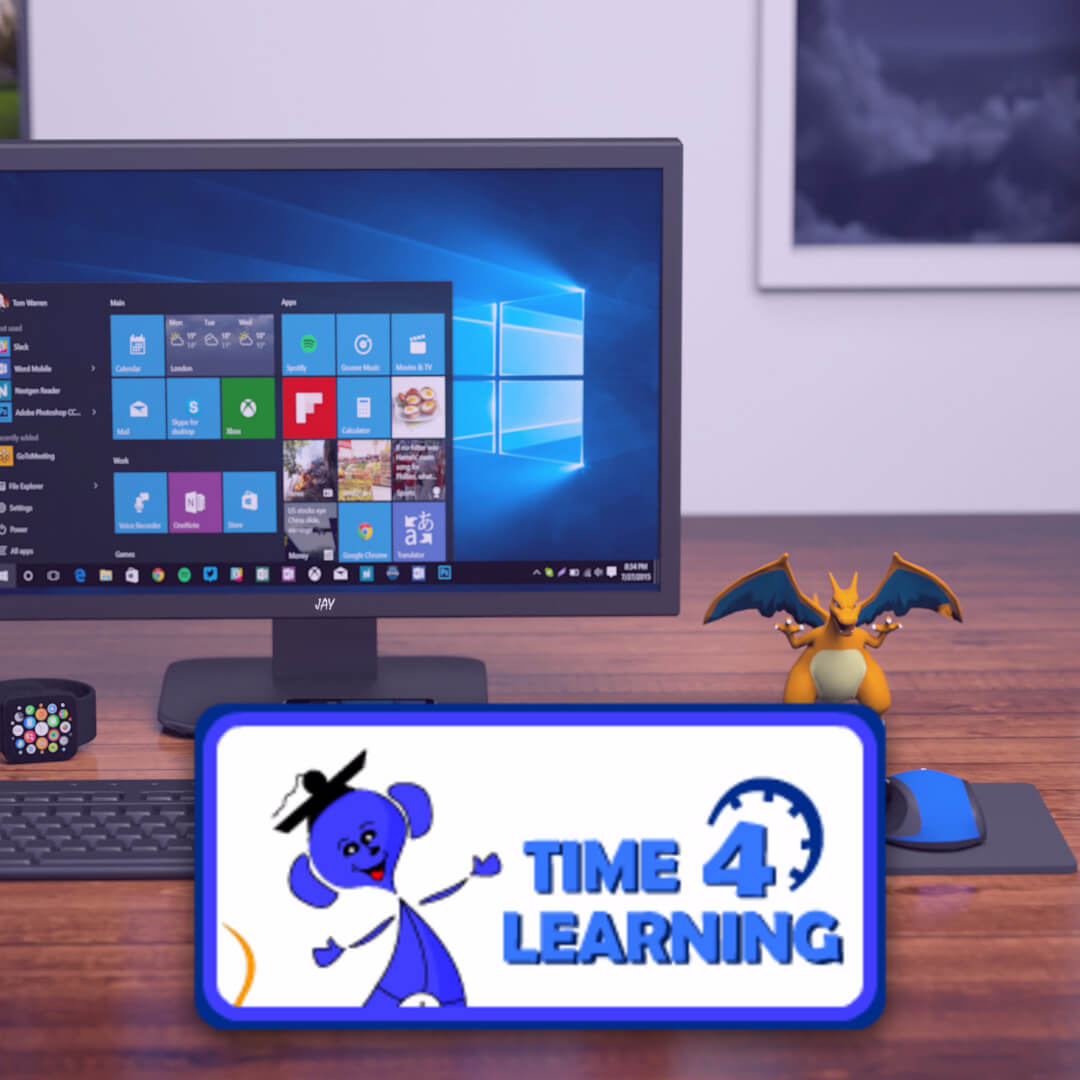## Time4Learning - Complete Online Curriculum

Time4Learning is a curriculum based online for all grade levels, K-12. Time4Learning is one of the most well known names in home education. But Time4Learning isn't just for homeschool families. It can...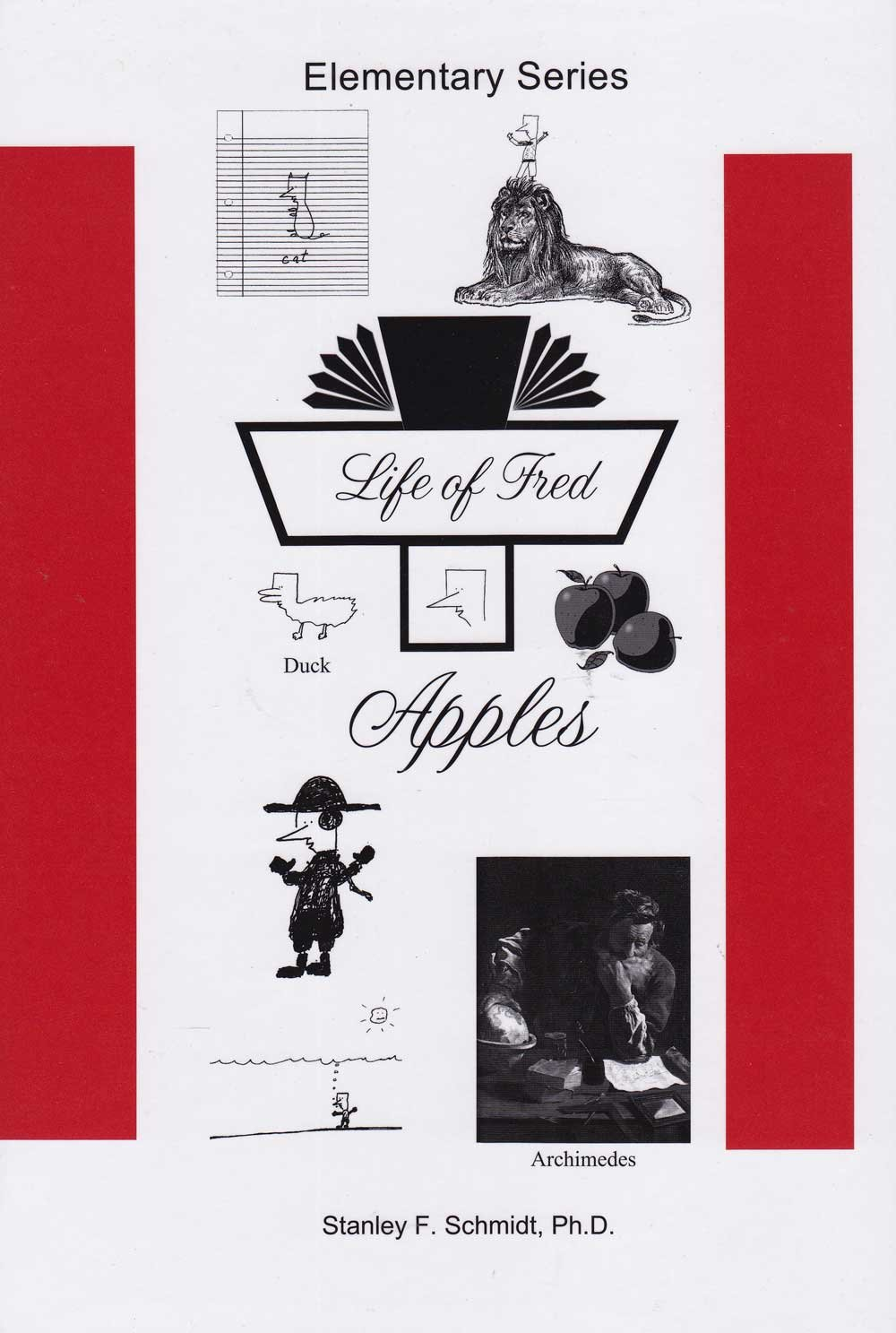## Life of Fred: Mathematics

Life of Fred Math math “Life of Fred” is quickly becoming a buzzword in the homeschooling world. If any child has ever struggled with understanding math concepts, Fred is ready to jump in and help you...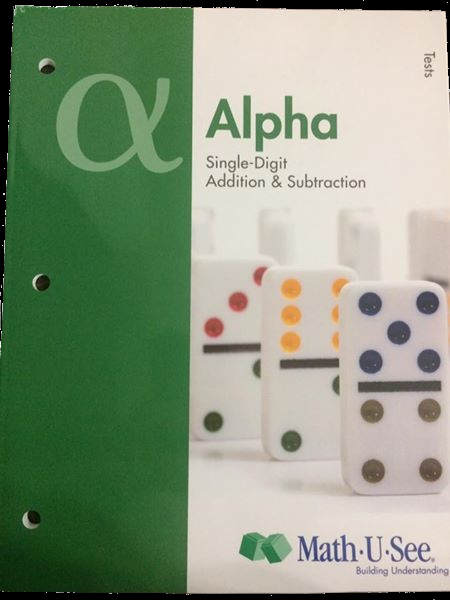## Math-U-See: Complete K-12 Math

Designed by a homeschool dad, Math-U-See is a manipulative-based math curriculum for K-12 that was specifically created with homeschoolers and small groups in mind. Math-U-See utilizes video instructi...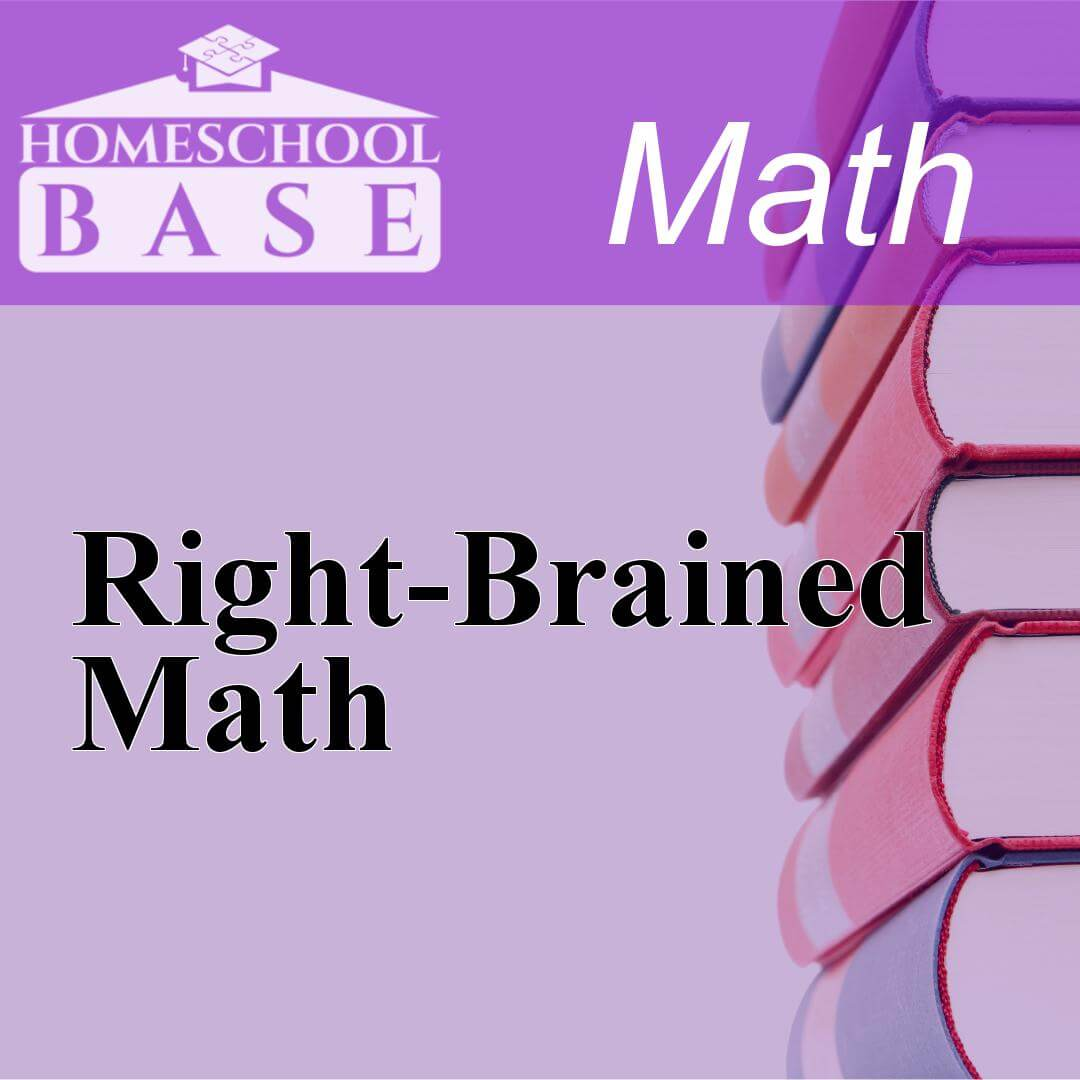## Right-Brained Math

Right-Brained Math is a Math curriculum for grades 2-7. This program teaches by using the conceptual-topical method. The average amount of teacher involvement on the parent(s) is: high. This curriculu...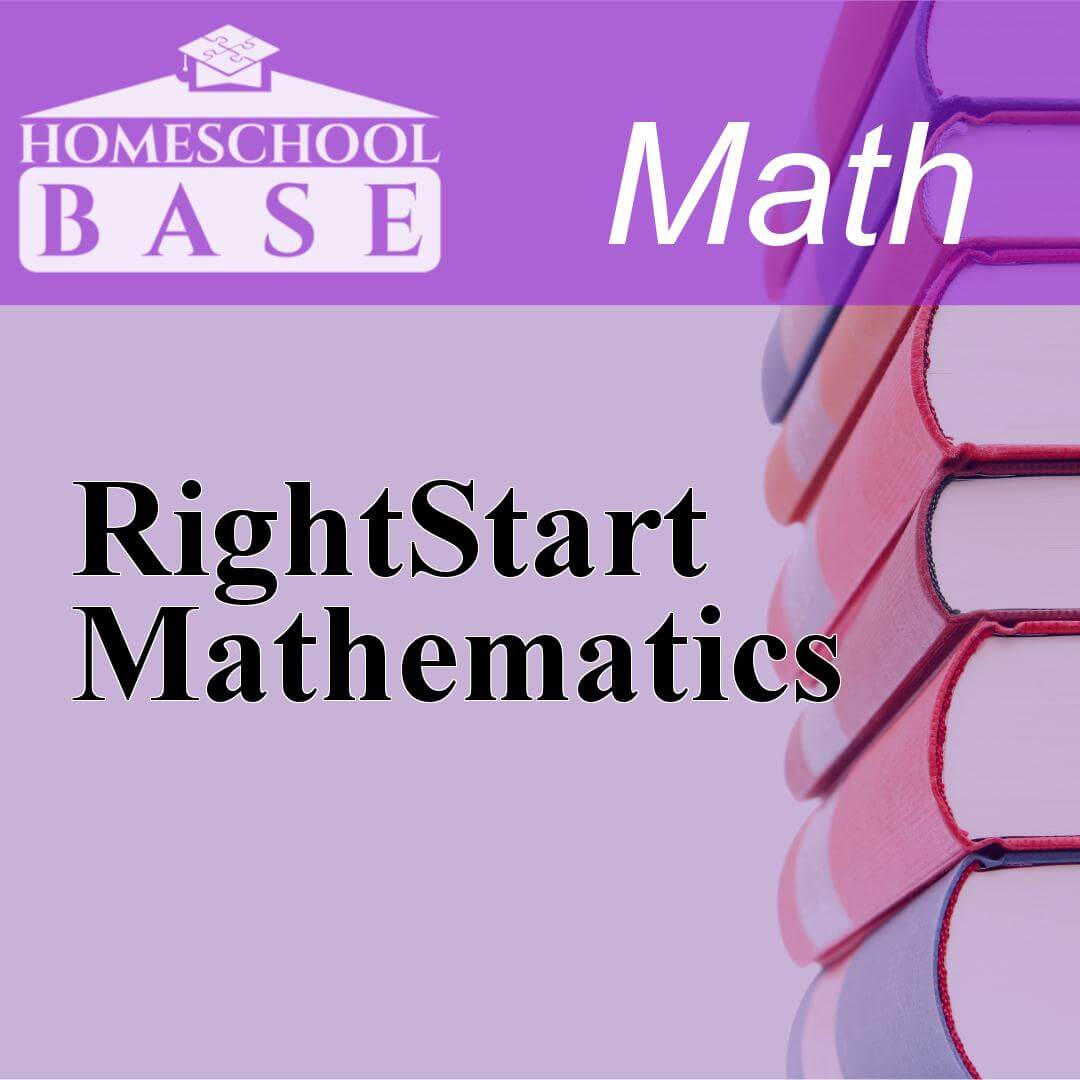## RightStart Mathematics

RightStart Mathematics is a Math curriculum for grades K-7. This program teaches by using the conceptual-topical method. The average amount of teacher involvement on the parent(s) is: high. This curr...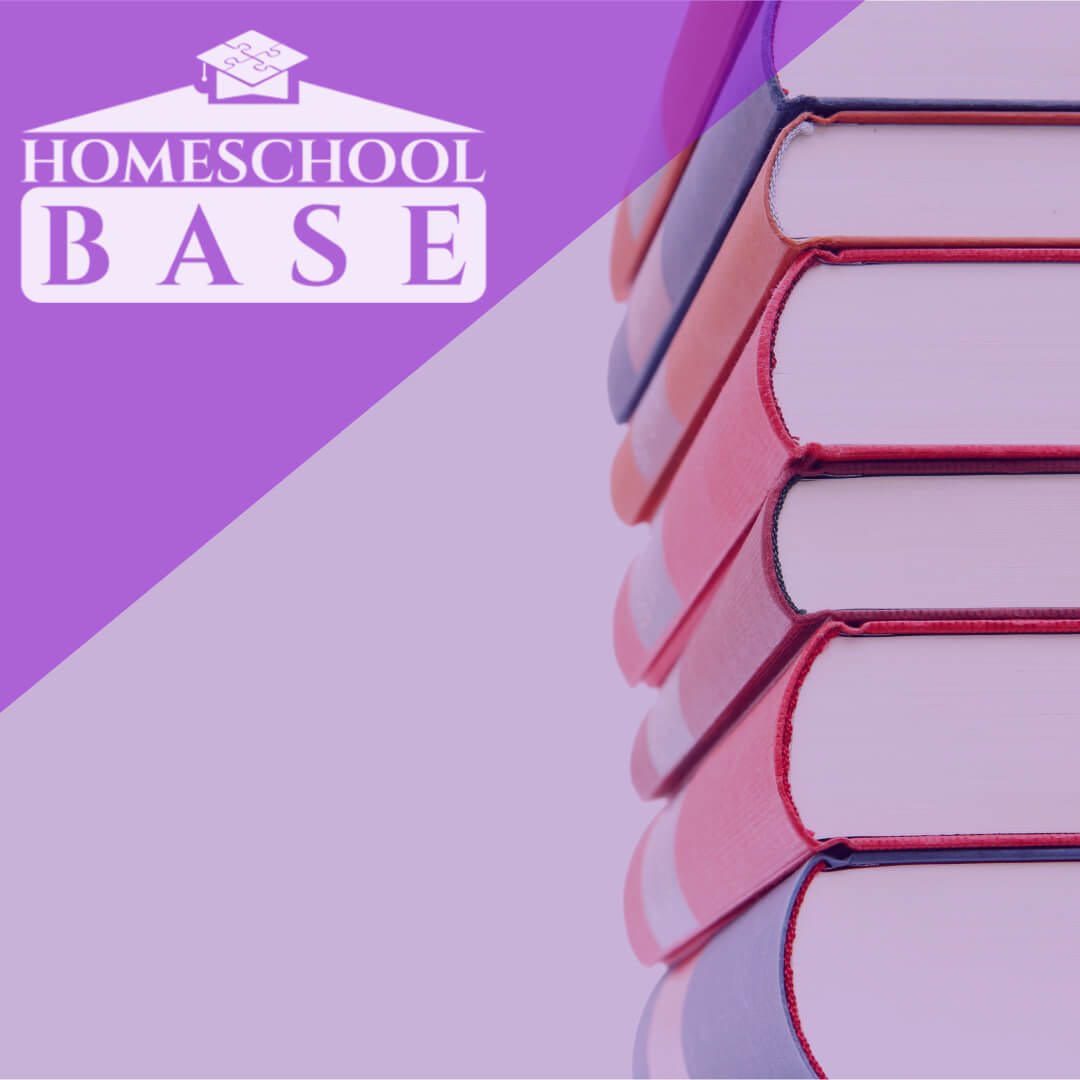## RightStart Mathematics

RightStart Mathematics is a Math curriculum for grades K,1,2,3,4,5,6,7. This program teaches by using the conceptual-topical method. The average amount of teacher involvement on the parent(s) is: hig...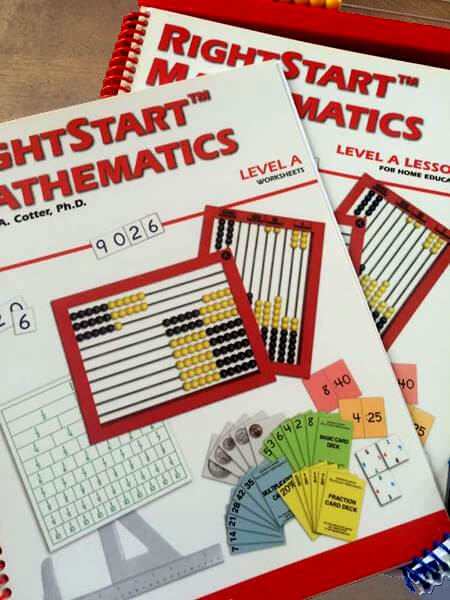## RightStart Mathematics

RightStart Math is the most unique math program on the market. It is inspired by the differences between Japanese classroom learning VS the U.S. RightStart uses many aspects of Asian math programs....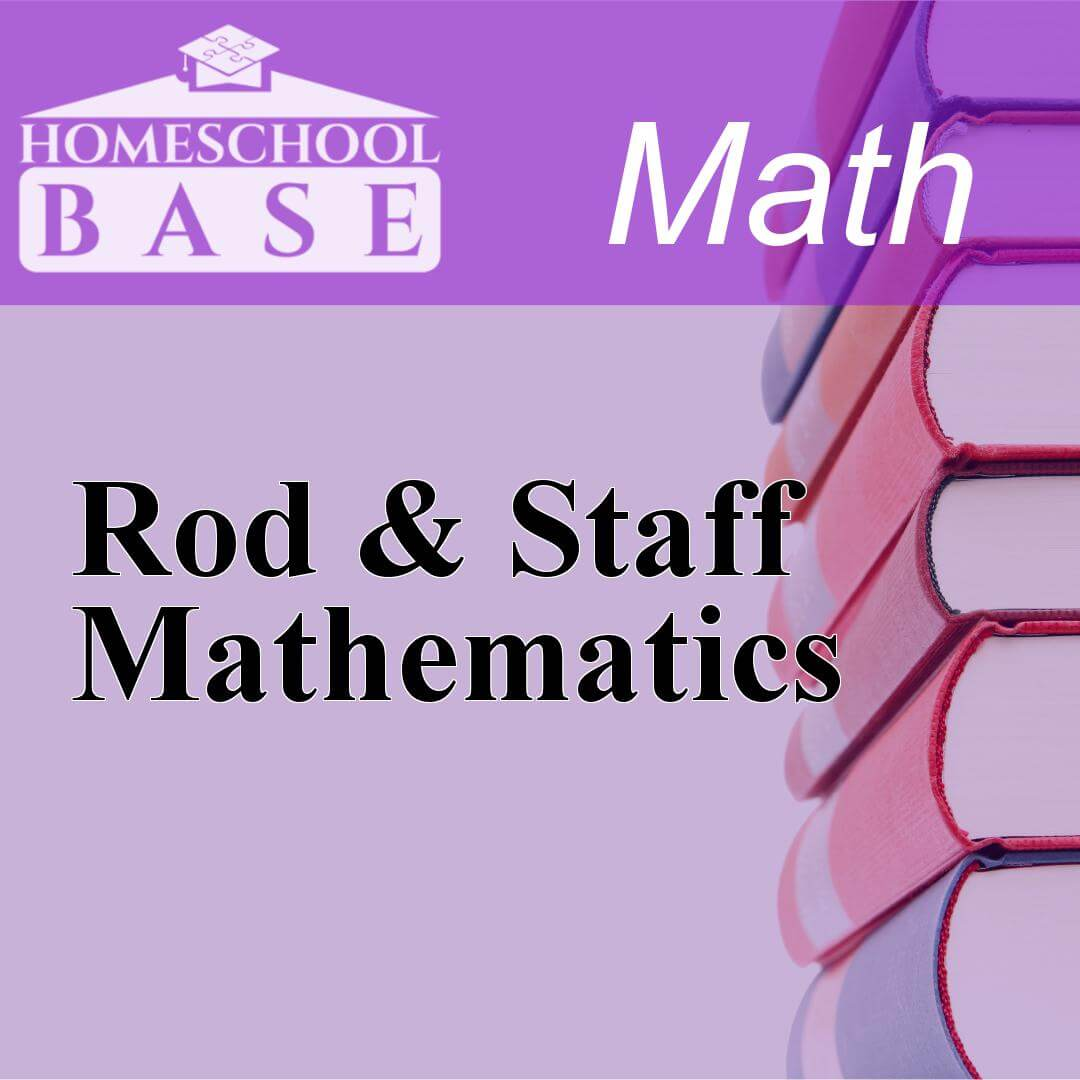## Rod & Staff Mathematics

Rod & Staff Mathematics is a Math curriculum for grades 1-8. This program teaches by using the spiral method. The average amount of teacher involvement on the parent(s) is: medium. This curriculum doe...